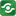Array Sorting@import url(http://www.google.com/cse/api/branding.css);CSGNetwork Custom SearchCSGNetwork Snippets Calculators Top Free Apps Home Back

Right click this window and select "view source" in order to copy the source for this script.

### Sorting an array in numerical order

For the most part, sorting numerical values means you want them sorted numerically. JavaScript understands that, and in a while, you will understand how to.

Sorting an array in numerical order still involves the same sort() method, with a little twist:

`arrayname.sort(functionname)`

"functionname" represents the returned value of a special function created specifically for the sort() method. A function like this has the following format:

```function myfunction(a,b){
return(a-b)
}```

Lets first put all this together and sort an array numerically, before anything else:

```function sortit(a,b){
return(a-b)
}
var myarray=new Array(70,43,8)
myarray.sort(sortit)
//myarray is now 8, myarray is now 43, myarray is now 70```

Ok, so what's "a" and "b", and why all this weird additional syntax? Well, "a" and "b" are two imaginary values the sort() method uses to determine how to sort an array. Subtracting "b" from "a"  indicates to the sort() method that we want it to sort the array numerically.

### Sorting an array in alphabetical order

The general syntax of the sort() method is as follows:

`arrayname.sort()`

The sort() method by default sorts the values inside an array in alphabetical order (dictionary order):

```var myarray=new Array("Bob","Bully","Amy")
myarray.sort()
//myarray is now Amy, myarray is now Bob, myarray is now Bully```

Before you starting wondering off, consider what happens if we sort numbers instead:

```var myarray=new Array(7,40)
myarray.sort()
//myarray is now 40, myarray is now 7```

Although 7 is numerically smaller than 40, alphabetically , it is larger, since 7 itself is larger than 4. The sort() method by default always treats values as strings and sorts them as such. If you're confused about the criteria the sort() method uses when sorting, simply flip through a dictionary. The way the words in a dictionary are indexed follows the same rule.

#### Example: Numbers and Words Sorter

Lets create a script now that sorts any collection of numbers or words entered and sort them.

Enter into the following text box "34 22 23 435 33 2 1 43 54", for example, and press the sort numerically button. Or, try entering "this is a test", and press the sort alpha button. The code for the script is below the text box.

```<script type="text/javascript">

function sortit(a,b){
return(a-b)
}

function sortvalues(param){
var inputvalues=document.sorter.sorter2.value.split(" ")
if (param==0) //if sort alphabetically
inputvalues.sort()
else //else if sort numerically
inputvalues.sort(sortit)
document.sorter.sorter2.value=''
for (i=0;i<inputvalues.length-1;i++)
document.sorter.sorter2.value=document.sorter.sorter2.value+inputvalues[i]+" "
document.sorter.sorter2.value+=inputvalues[inputvalues.length-1]
}
</script>

<form name="sorter">
<p>
<textarea rows="10" name="sorter2" cols="50" wrap="virtual"></textarea><br>
<input type="button" value="Sort alphabetically" onClick="sortvalues(0)">
<input type="button" value="Sort numerically" onClick="sortvalues(1)">
<input type="reset" value="Reset">
</form>```